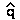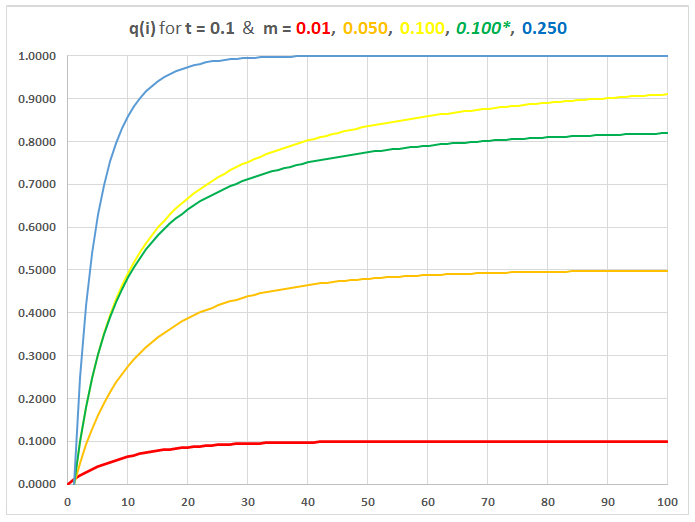Migration (Gene Flow) / Selection equilibrium

Consider an island adjacent to a mainland, with unidirectional migration from the mainland to the island.
The mainland is uniformly dark, the island uniformly light
AA
phenotypes are light, BB dark, and AB intermediate

Phenotypes are subject to selection according to degree of crypsis

Fitness of AA, AB, & BB genotypes differ between environments,
so allele frequency q = f(B) differs between mainland (qm) and island (qi)
B has high fitness on mainland, low fitness on island

For this model, A semi-dominant to B:
B
: WAB = (1 - t)    WBB = (1 - 2t)

[Note: use t so as not to confuse additive vs recessive s]

 WAA WAB WBB qinit Island 1 1 - t 1 - 2t qi0 Mainland 0 0 1 qm1

Migration rate m = fraction of island population newly arrived from mainland
[m equivalent to fraction of new alleles arriving from mainland]

Migration / Selection equilibria resemble Mutation / Selection equilibria mathematically, except
Migration introduces alleles two at a time (BB diploid migrants vs AB gametic mutations)
Migration rates m >> Mutation rates u

Calculate equilibrium frequency whereqi =f(B) = 0 on island
Change in f(B) from migrationqi = m(qm - qi)
Change in f(B) from selectionqi  = -tqi(1 - qi)) / (1 - 2tqi)-tqi(1 - qi)                          [if tqi << 1]
Then, combined changeqi = m(qm - qi) - tqi(1 - qi)
= mqm - mqi - tqi + tqi2)
= tqi2 - (m + t)(qi) + mqm

Forqi = 0   solve as quadratic equation for several special cases:

ift:      qiqm              migration behaves like mutation
[except: alleles introduced at higher rate, as diploids]

if m >> t:      qiqm                 mainland B allele 'swamps' island A allele

if m << t:      qi(m / t)(qm)     some equilibriumachieved, iff m constant

Intermediate cases can be simulated in GSM worksheetApproach to Mutation-Selection Equilibriumwhereqi = 0
for t = 0.1 & m = 0.01 ~ 0.250

For m = 0.250, qI rapidly approaches gM: migration swamps locally favored allele, prevents local adaptation.

For m = 0.01 or 0.05, qI reaches equilibrium at (m / t) as predicted: deleterious mainland allele maintained at relatively high frequency.

For m = 0.1 = t,
qI approaches qM but very slowly. The exact solution with m = 0.1* flattens earlier.

Text material © 2021 by Steven M. Carr